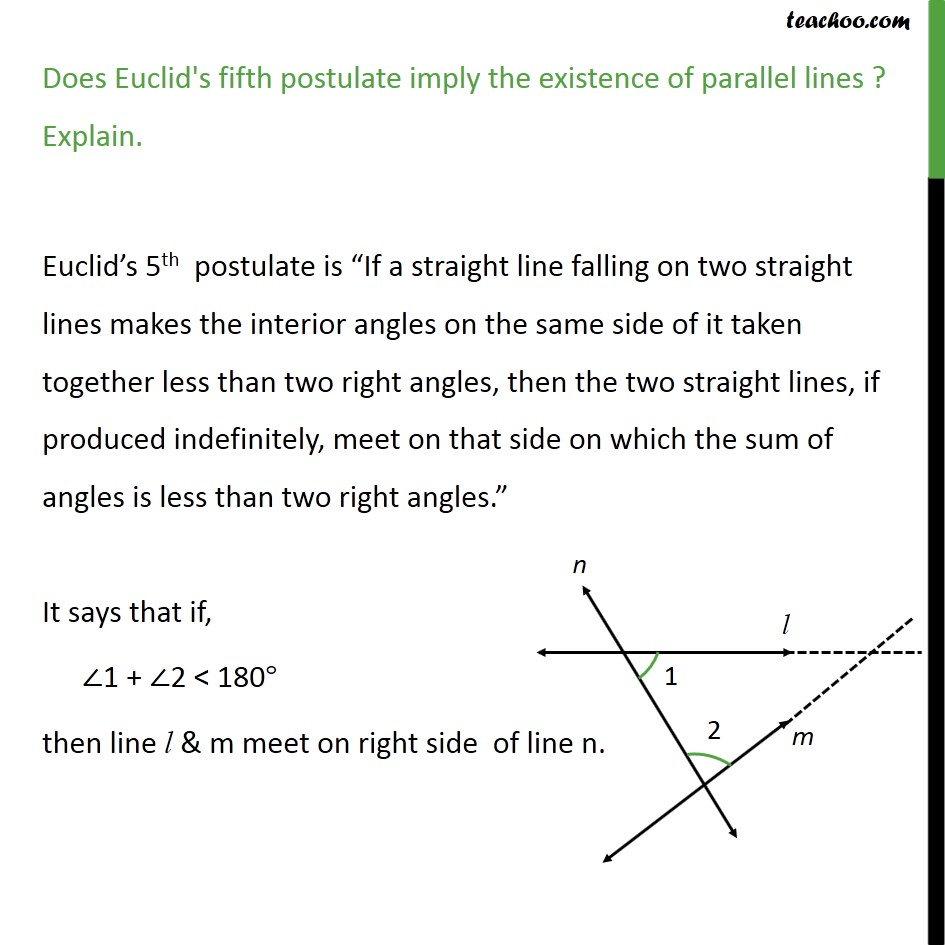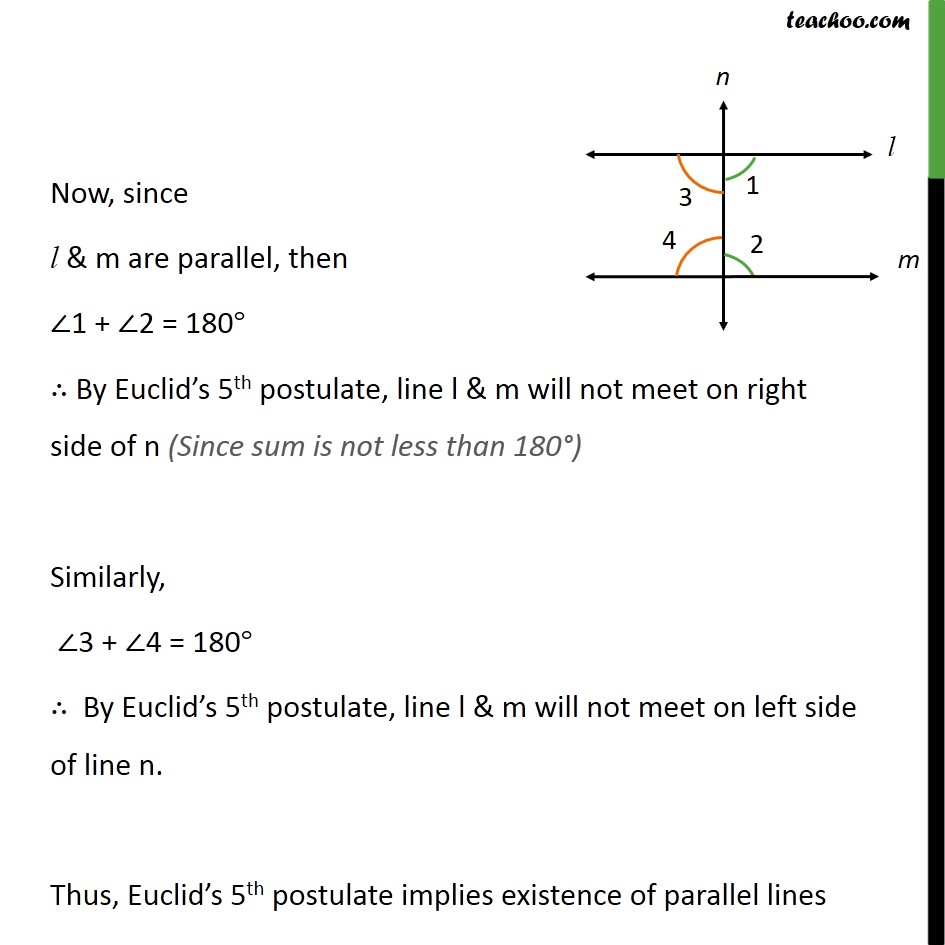Subscribe to our Youtube Channel - https://you.tube/teachoo

1. Chapter 5 Class 9 Introduction to Euclid's Geometry
2. Concept wise
3. Postulates

Transcript

Ex 5.2, 2 Does Euclid s fifth postulate imply the existence of parallel lines? Explain. Postulate 5 : If a straight line falling on two straight lines makes the interior angles on the same side of it taken together less than two right angles, then the two straight lines, if produced indefinitely, meet on that side on which the sum of angles is less than two right angle If a straight line PQ falls on two straight lines AB & CD such that 1 + 2 = 180 & 1 = 90 , 2 = 90 Then lines AB & CD wont meet, So, lines AB & CD are parallel.

Postulates